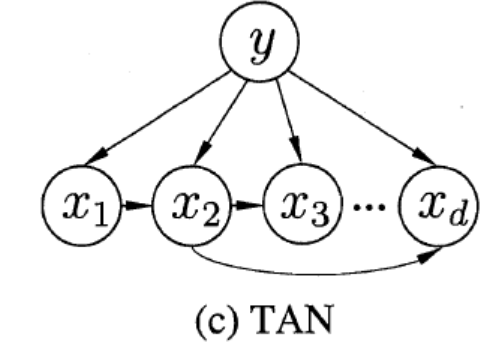# 引言

$P\left(B_{i} \mid A\right)=\frac{P\left(A \mid B_{i}\right) P\left(B_{i}\right)}{\sum_{j=1}^{n} P\left(A \mid B_{j}\right) P\left(B_{j}\right)}, \quad i=1,2, \cdots, n$

$P\left(C \mid \boldsymbol x\right)=\frac{P\left(\boldsymbol x \mid C\right) P\left(C\right)}{\sum_{j=1}^{n} P\left(\boldsymbol x \mid C\right) P\left(C\right)}, \quad i=1,2, \cdots, n$

# 贝叶斯决策论

$R\left(c_{i} \mid \boldsymbol{x}\right)=\sum_{j=1}^{N} \lambda_{i j} P\left(c_{j} \mid \boldsymbol{x}\right)$

## 基于最小风险的贝叶斯决策

$R(h)=\mathbb{E}_{\boldsymbol{x}}[R(h(\boldsymbol{x}) \mid \boldsymbol{x})]$

$h^{*}(\boldsymbol{x})=\underset{c \in \mathcal{Y}}{\arg \min } R(c \mid \boldsymbol{x})$

## 基于最小错误率的贝叶斯决策

$\lambda_{i j}=\left\{\begin{array}{ll} 0, & \text { if } i=j \\ 1, & \text { otherwise } \end{array}\right.$

$R(c \mid \boldsymbol{x})=1-P(c \mid \boldsymbol{x})$

$h^{*}(\boldsymbol{x})=\underset{c \in \mathcal{Y}}{\arg \max } P(c \mid \boldsymbol{x})$

# 朴素贝叶斯分类器

$P(c \mid \boldsymbol{x})=\frac{P(c) P(\boldsymbol{x} \mid c)}{P(\boldsymbol{x})}=\frac{P(c)}{P(\boldsymbol{x})} \prod_{i=1}^{d} P\left(x_{i} \mid c\right)$

$h_{n b}(\boldsymbol{x})=\underset{c }{\arg \max } P(c) \prod_{i=1}^{d} P\left(x_{i} \mid c\right)$

$P(c)=\frac{\left|D_{c}\right|}{|D|}$

1. 对于离散属性
$D_{c,xi}$表示Dc中在第闪属性上取值为$x_i$的样本组成的集合, 则条件概率可估计为
$P\left(x_{i} \mid c\right)=\frac{\left|D_{c, x_{i}}\right|}{\left|D_{c}\right|}$
2. 对于连续属性
可考虑概率密度函数，假定服从正态分布, 则有:
$p\left(x_{i} \mid c\right)=\frac{1}{\sqrt{2 \pi} \sigma_{c, i}} \exp \left(-\frac{\left(x_{i}-\mu_{c, i}\right)^{2}}{2 \sigma_{c, i}^{2}}\right)$

\begin{aligned} \hat{P}(c) &=\frac{\left|D_{c}\right|+1}{|D|+N} \\ \hat{P}\left(x_{i} \mid c\right) &=\frac{\left|D_{c, x_{i}}\right|+1}{\left|D_{c}\right|+N_{i}} \end{aligned}

# 半朴素贝叶斯分类器

\begin{aligned} P(y \mid x) &=\frac{P(y) P(x \mid y)}{P(x)} =\frac{P(y)}{P(x)} \prod_{i=1}^{d} P\left(x_{i} \mid x_{ik}, y\right) \end{aligned}

1. 超父独依赖估计(Super-Parent ODE,SPODE)
这个方法的含义是选择一个特征作为其他所有特征所依赖的特征，称为超父。一般会通过交叉验证等方法确定。
2. TAN(Tree Augmented naive Bayes):
这种方法的基础是最大带权生成树，即(1). 首先计算每两个特征之间的条件互信息；(2). 然后以特征为节点，以特征之间的条件互信息作为权值来构建一个完全图，根据该完全图再构建一个最大带权生成树，挑选根变量，将边置为有向；(3). 最后再加入y，增加从y到每个特征的有向边。其结构如下图所示：条件互信息的计算方法如下
$I\left(x_{i}, x_{j} \mid y\right)=\sum_{x_{i}, x_{j} ; y \in Y} P\left(x_{i}, x_{j} \mid y\right) \log \frac{P\left(x_{i}, x_{j} \mid y\right)}{P\left(x_{i} \mid y\right) P\left(x_{j} \mid y\right)}$
3. AODE(Average One-Dependent Estimator)
是一种基于集成学习机制的独依赖分类器，它将每个属性作为超父来构建SPODE，然后将那些具有足够训练数据支撑的SPODE集成起来作为最终结果。

11-26

#### 贝叶斯分类器

02-10#### 机器学习之贝叶斯分类器视频教学

01-15

05-225458

#### 机器学习笔记之（2）——贝叶斯分类器

02-121104

#### 机器学习笔记 贝叶斯分类器（附Python与R代码）

04-12625

#### 小白学习机器学习---第七章:贝叶斯分类器

09-0253

#### 《机器学习》 周志华学习笔记第七章 贝叶斯分类器（课后习题）python 实现

03-25243

#### 机器学习笔记(五)——轻松看透朴素贝叶斯

05-211260

#### 读书笔记-《机器学习》第七章：贝叶斯分类器©️2020 CSDN 皮肤主题: 数字20 设计师: CSDN官方博客点击重新获取扫码支付1.余额是钱包充值的虚拟货币，按照1:1的比例进行支付金额的抵扣。
2.余额无法直接购买下载，可以购买VIP、C币套餐、付费专栏及课程。余额充值